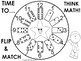# Grade One Math Games~ A Bundle of Hands-On FunSubject
Resource Type
File Type
PDF (8 MB|72 pages)
Standards
\$6.99
• Product Description
• Standards

This is the ultimate package of PRINTABLE primary math games that will keep you and your students busy all year long.

Hands-on games and activities cover a range of common core standards:

* Number recognition

* Subitizing

* Graphing : Colors/Shapes/Numbers/Tallying/Color Words/Number

Words/ Number Rhyming Words

* Clocks and beginning Telling Time

* Addition to 12/ Fact Families

* Subtraction

* Counting

* Problem Solving

* Social Game Playing Skills

* Following Directions and MORE!

The variety of games and easy game rules with Teaching Tips makes this a wonderful and useful resource for any primary classroom.

Your "Little Geniuses" will love these engaging, interactive games and so will you!

Determine the unknown whole number in an addition or subtraction equation relating three whole numbers. For example, determine the unknown number that makes the equation true in each of the equations 8 + ? = 11, 5 = ▯ - 3, 6 + 6 = ▯.
Understand the meaning of the equal sign, and determine if equations involving addition and subtraction are true or false. For example, which of the following equations are true and which are false? 6 = 6, 7 = 8 - 1, 5 + 2 = 2 + 5, 4 + 1 = 5 + 2.
Add and subtract within 20, demonstrating fluency for addition and subtraction within 10. Use strategies such as counting on; making ten (e.g., 8 + 6 = 8 + 2 + 4 = 10 + 4 = 14); decomposing a number leading to a ten (e.g., 13 - 4 = 13 - 3 - 1 = 10 - 1 = 9); using the relationship between addition and subtraction (e.g., knowing that 8 + 4 = 12, one knows 12 - 8 = 4); and creating equivalent but easier or known sums (e.g., adding 6 + 7 by creating the known equivalent 6 + 6 + 1 = 12 + 1 = 13).
Relate counting to addition and subtraction (e.g., by counting on 2 to add 2).
Understand subtraction as an unknown-addend problem. For example, subtract 10 – 8 by finding the number that makes 10 when added to 8.
Total Pages
72 pages